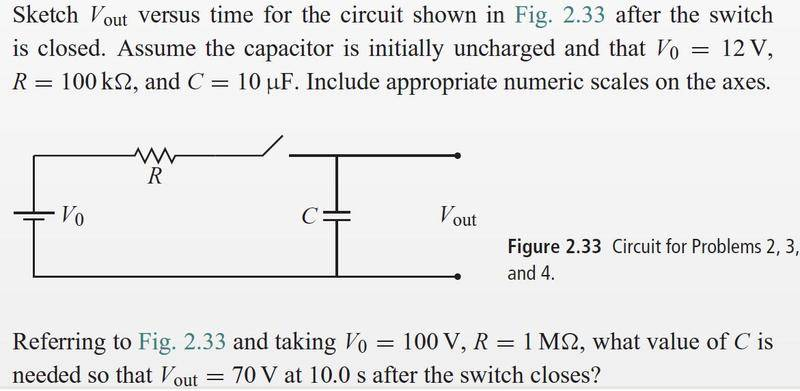# RC circuits voltage

Dens

## Homework StatementRelevant infor
V(out) stands for the voltage divider I believe

## The Attempt at a Solution

I am just going to get the equation first.

For the first part, applying the loop rule I got

$$12 - 10^5q' - 10^7 q = 0$$ with q(0) = 0. Solving I get

$$Q = \frac{3}{25000}(1 - e^{-100t})$$

Since V = IR = q'R = q'(105) I should get $$V = 12e^{-100t}$$. So the plot would be a exp curve, curving down.

For the question that follows after. The loop rule is

$$100 - q'(10^6) - q/C = 0$$. Solving, I get

$$q(t) = 100C - 100Ce^{-10^{-6}t}$$

Since V = IR = q'R = q'(106) I should get $$V = 100e^{-10^{-6}t/C}$$

Now $$V_{out} = 70 = 100e^{-10^{-5}/C}$$, solving I get C = 2.8

Here is the problem, the answer is supposed to be 8.3μF. All the ODEs were solved with Maple

What did I do wrong?

Staff Emeritus
Gold Member
Relevant infor
V(out) stands for the voltage divider I believe

Huh?What voltage divider? Vout is the output voltage, which is the voltage across the capacitor, in this circuit.

## The Attempt at a Solution

I am just going to get the equation first.

For the first part, applying the loop rule I got

$$12 - 10^5q' - 10^7 q = 0$$ with q(0) = 0. Solving I get

$$Q = \frac{3}{25000}(1 - e^{-100t})$$

Since V = IR = q'R = q'(105) I should get $$V = 12e^{-100t}$$. So the plot would be a exp curve, curving down.

You're solving for the WRONG V here. The voltage given by IR is the voltage across the resistor (let's call it VR). You're looking for the voltage across the capacitor. To get the capacitor voltage (VC), use the fact that q = CVC for a capacitor. OR, use the loop rule to solve for VC in terms of VR and V0. Either method should give you the same answer.

Also, whenever you get an answer to a problem, always ask yourself, "does this make any sense?" A decaying exponential for the capacitor voltage makes no sense, because after the switch closes, the capacitor is charging, not discharging.EDIT: and you made the exact same mistake in part 2. Your equation for the capacitor voltage vs. time is just wrong.